# MISC#

## Welcome#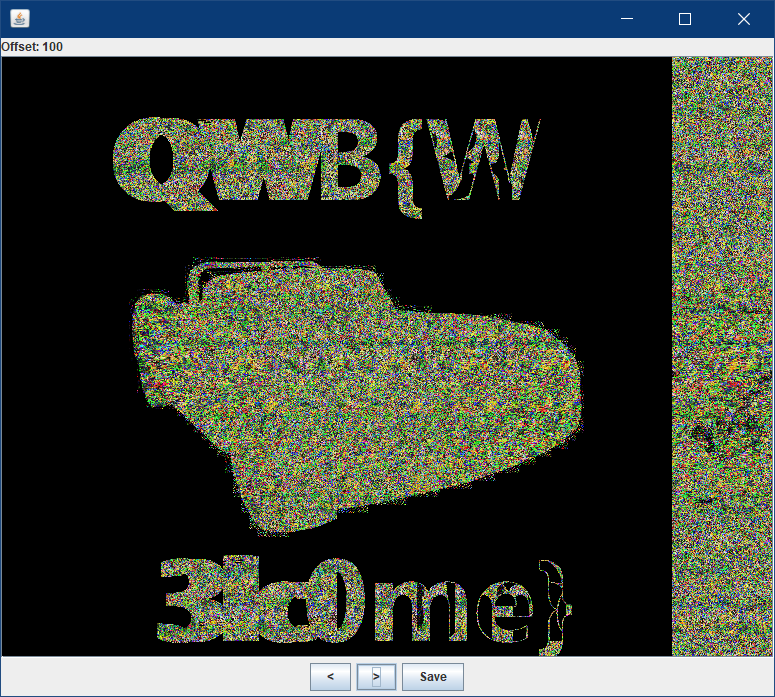# Crypto#

## streamgame1#

streamgame1.py

 `````` 1 2 3 4 5 6 7 8 9 10 11 12 13 14 15 16 17 18 19 20 21 22 23 24 25 26 27 `````` ``````from flag import flag assert flag.startswith("flag{") assert flag.endswith("}") assert len(flag)==25 def lfsr(R,mask): output = (R << 1) & 0xffffff i=(R&mask)&0xffffff lastbit=0 while i!=0: lastbit^=(i&1) i=i>>1 output^=lastbit return (output,lastbit) R=int(flag[5:-1],2) mask = 0b1010011000100011100 f=open("key","ab") for i in range(12): tmp=0 for j in range(8): (R,out)=lfsr(R,mask) tmp=(tmp << 1)^out f.write(chr(tmp)) f.close() ``````

solution

 `````` 1 2 3 4 5 6 7 8 9 10 11 12 13 14 15 16 `````` ``````def crack(key): for k in range(0,2**19): R=k for i in range(12): tmp,flag=0,1 for j in range(8): (R,out)=lfsr(R,mask) tmp=(tmp << 1)^out if(chr(tmp)!=key[i]): flag=0 break if flag: print "flag{%s}"%bin(k)[2:] crack(open('key','rb').read()) # flag{1110101100001101011} ``````

## streamgame2#

streamgame2.py

 `````` 1 2 3 4 5 6 7 8 9 10 11 12 13 14 15 16 17 18 19 20 21 22 23 24 25 26 `````` ``````from flag import flag assert flag.startswith("flag{") assert flag.endswith("}") assert len(flag)==27 def lfsr(R,mask): output = (R << 1) & 0xffffff i=(R&mask)&0xffffff lastbit=0 while i!=0: lastbit^=(i&1) i=i>>1 output^=lastbit return (output,lastbit) R=int(flag[5:-1],2) mask=0x100002 f=open("key","ab") for i in range(12): tmp=0 for j in range(8): (R,out)=lfsr(R,mask) tmp=(tmp << 1)^out f.write(chr(tmp)) f.close() ``````

solution

 `````` 1 2 3 4 5 6 7 8 9 10 11 12 13 14 15 16 `````` ``````def crack(key): for k in range(0,2**21): R=k for i in range(12): tmp=0 flag=1 for j in range(8): (R,out)=lfsr(R,mask) tmp=(tmp << 1)^out if(chr(tmp)!=key[i]): flag=0 break if flag: print "flag{%s}"%bin(k)[2:] crack(open('key','rb').read()) # flag{110111100101001101001} ``````

## streamgame3#

 `````` 1 2 3 4 5 6 7 8 9 10 11 12 13 14 15 16 17 18 19 20 21 22 23 24 25 26 27 28 29 30 31 32 33 34 35 36 37 38 39 40 41 42 43 44 45 46 47 48 49 50 51 52 53 54 55 56 57 `````` ``````from flag import flag assert flag.startswith("flag{") assert flag.endswith("}") assert len(flag)==24 def lfsr(R,mask): output = (R << 1) & 0xffffff i = (R&mask)&0xffffff lastbit=0 while i!=0: lastbit^=(i&1) i=i>>1 output^=lastbit return (output,lastbit) def single_round(R1,R1_mask,R2,R2_mask,R3,R3_mask): (R1_NEW,x1)=lfsr(R1,R1_mask) (R2_NEW,x2)=lfsr(R2,R2_mask) (R3_NEW,x3)=lfsr(R3,R3_mask) return (R1_NEW, R2_NEW, R3_NEW, (x1*x2)^((x2^1)*x3) ) R1=int(flag[5:11],16) R2=int(flag[11:17],16) R3=int(flag[17:23],16) assert len(bin(R1)[2:])==17 assert len(bin(R2)[2:])==19 assert len(bin(R3)[2:])==21 R1_mask=0x10020 R2_mask=0x4100c R3_mask=0x100002 for fi in range(1024): print fi tmp1mb="" for i in range(1024): tmp1kb="" for j in range(1024): tmp=0 for k in range(8): (R1,R2,R3,out)=single_round(R1,R1_mask,R2,R2_mask,R3,R3_mask) tmp = (tmp << 1) ^ out tmp1kb+=chr(tmp) tmp1mb+=tmp1kb f = open("./output/" + str(fi), "ab") f.write(tmp1mb) f.close() ``````

solution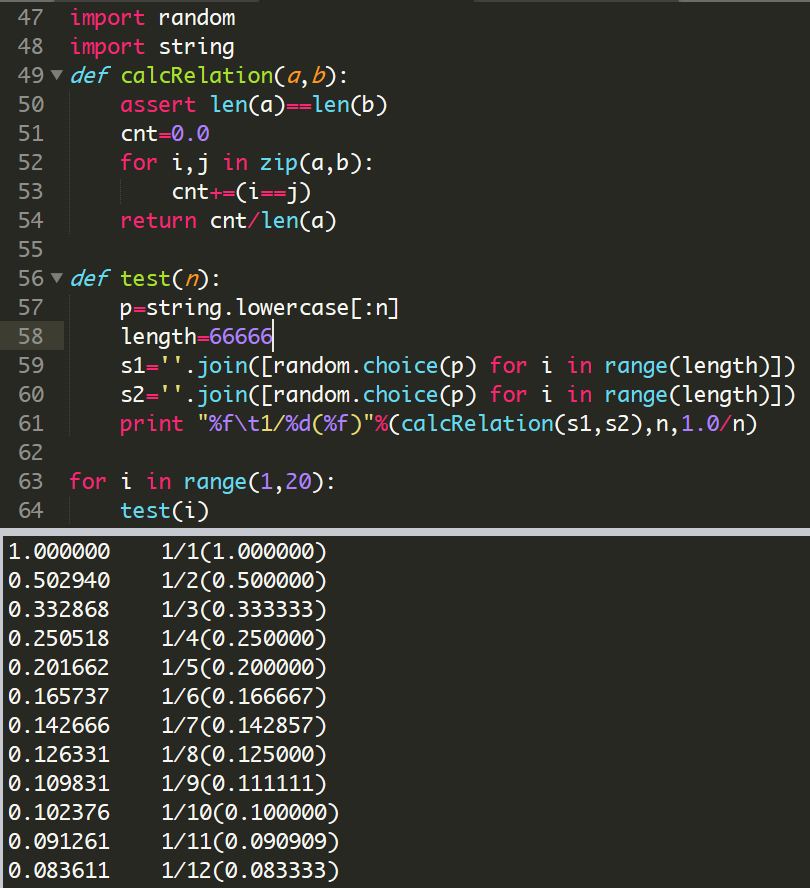`x2=0` ，则`out=x3` ，若`x2=1``out=x1`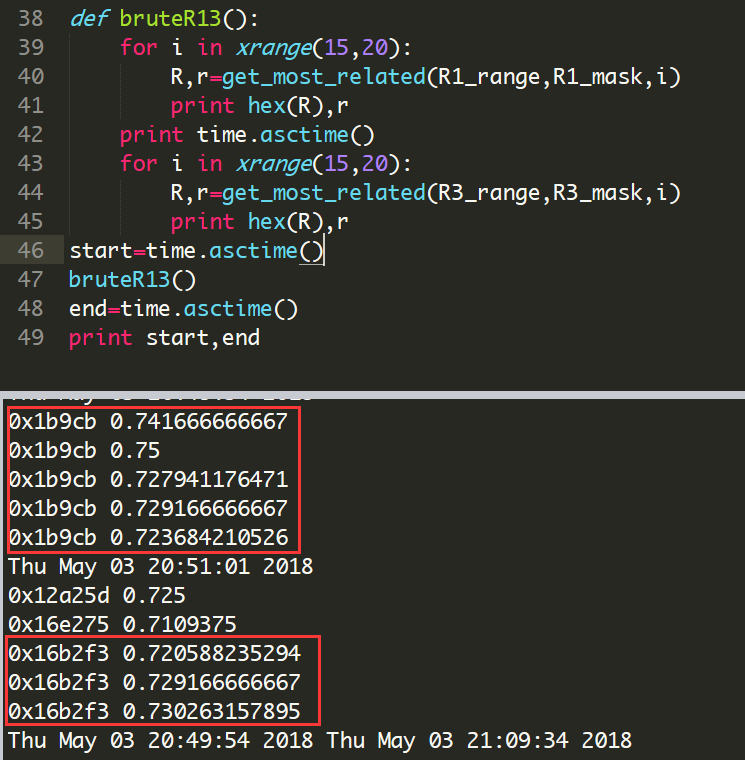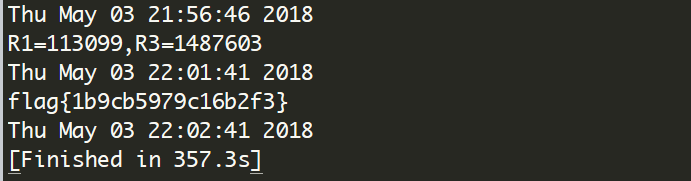`````` 1 2 3 4 5 6 7 8 9 10 11 12 13 14 15 16 17 18 19 20 21 22 23 24 25 26 27 28 29 30 31 32 33 34 35 36 37 38 39 40 41 42 43 44 45 46 47 48 49 50 51 52 53 54 55 56 57 58 59 60 61 62 63 64 65 66 67 68 69 70 71 72 `````` ``````import time cipher=''.join([bin(ord(i))[2:].zfill(8) for i in open("output/0","rb").read()]) R1_mask=0x10020 ; R2_mask=0x4100c ; R3_mask=0x100002 R1_range=xrange(2**16,2**17) R2_range=xrange(2**18,2**19) R3_range=xrange(2**20,2**21) def lfsr(R,mask): output = (R << 1) & 0xffffff i=(R&mask)&0xffffff lastbit=0 while i!=0: lastbit^=(i&1) i=i>>1 output^=lastbit return (output,lastbit) def single_round(R1,R1_mask,R2,R2_mask,R3,R3_mask): (R1_NEW,x1)=lfsr(R1,R1_mask) (R2_NEW,x2)=lfsr(R2,R2_mask) (R3_NEW,x3)=lfsr(R3,R3_mask) return (R1_NEW, R2_NEW, R3_NEW, (x1*x2)^((x2^1)*x3) ) def calcRelation(a,b): assert len(a)==len(b) cnt=0.0 for i,j in zip(a,b): cnt+=(i==j) return cnt/len(a) def get_most_related(R_range,R_mask,cmp_length): real_R,relation=0,0 for i in R_range: R,tmp=i,"" for j in xrange(cmp_length*8): R,out=lfsr(R,R_mask) tmp+=str(out) r=calcRelation(cipher[:cmp_length*8],tmp) if r>relation: relation=r real_R=i return (real_R,relation) def bruteR13(): R1,r=get_most_related(R1_range,R1_mask,7) R3,r=get_most_related(R3_range,R3_mask,17) return (R1,R3) def brute(): R1_,R3_=bruteR13() # R1_,R3_=0x1b9cb,0x16b2f3 print "R1=%s,R3=%s"%(R1_,R3_) print time.asctime() for i in R2_range: R1,R2,R3=R1_,i,R3_ res='' for j in range(40): (R1,R2,R3,out)=single_round(R1,R1_mask,R2,R2_mask,R3,R3_mask) res+=str(out) if cipher[:len(res)]==res: print 'flag{'+''.join(map(lambda x:hex(x)[2:],(R1_,i,R3_)))+'}' print time.asctime() brute() print time.asctime() # Thu May 03 21:56:46 2018 # R1=113099,R3=1487603 # Thu May 03 22:01:41 2018 # flag{1b9cb5979c16b2f3} # Thu May 03 22:02:41 2018 # [Finished in 357.3s] ``````

## streamgame4#

streamgame4.py

 `````` 1 2 3 4 5 6 7 8 9 10 11 12 13 14 15 16 17 18 19 20 21 22 23 24 25 26 27 28 29 30 31 `````` ``````from flag import flag assert flag.startswith("flag{") assert flag.endswith("}") assert len(flag)==27 def nlfsr(R,mask): output = (R << 1) & 0xffffff i=(R&mask)&0xffffff lastbit=0 changesign=True while i!=0: if changesign: lastbit &= (i & 1) changesign=False else: lastbit^=(i&1) i=i>>1 output^=lastbit return (output,lastbit) R=int(flag[5:-1],2) mask=0b110110011011001101110 f=open("key","ab") for i in range(1024*1024): tmp=0 for j in range(8): (R,out)=nlfsr(R,mask) tmp=(tmp << 1)^out f.write(chr(tmp)) f.close() ``````

solution

 `````` 1 2 3 4 5 6 7 8 9 10 11 12 13 14 15 16 17 `````` ``````def crack(key): for maybe in range(2**21,0,-1): flag=0 R=maybe for index in xrange(len(key)): tmp=0 for j in range(8): (R,out)=nlfsr(R,mask) tmp=(tmp<<1)^out if chr(tmp)!=key[index]: flag=1 break if not flag: print "flag{%s}"%bin(maybe)[2:] crack(open("key",'r').read()) #flag{100100111010101101011} ``````

## nextrsa#

nc 39.107.33.90 9999

# Web#

## Web签到#

### The Fisrt Easy Md5 Challenge#

 ``````1 2 3 `````` ``````if(\$_POST['param1']!=\$_POST['param2'] && md5(\$_POST['param1'])==md5(\$_POST['param2'])){ die("success!"); } ``````

post `param1=QNKCDZO&param2=240610708` 或者 `param1[]=&param2[]=0`

### The Second Easy Md5 Challenge#

 ``````1 2 3 `````` ``````if(\$_POST['param1']!==\$_POST['param2'] && md5(\$_POST['param1'])===md5(\$_POST['param2'])){ die("success!"); } ``````

### Md5 Revenge Now!#

 ``````1 2 3 `````` ``````if((string)\$_POST['param1']!==(string)\$_POST['param2'] && md5(\$_POST['param1'])===md5(\$_POST['param2'])){ die("success!); } ``````

https://crypto.stackexchange.com/questions/1434/are-there-two-known-strings-which-have-the-same-md5-hash-value

 ``````1 2 3 4 5 `````` ``````s1="4dc968ff0ee35c209572d4777b721587d36fa7b21bdc56b74a3dc0783e7b9518afbfa200a8284bf36e8e4b55b35f427593d849676da0d1555d8360fb5f07fea2" s2="4dc968ff0ee35c209572d4777b721587d36fa7b21bdc56b74a3dc0783e7b9518afbfa202a8284bf36e8e4b55b35f427593d849676da0d1d55d8360fb5f07fea2" y=lambda s: "%"+"%".join([s[i*2:i*2+2] for i in range(len(s)/2)]) print y(s1) print y(s2) ``````
 `````` 1 2 3 4 5 6 7 8 9 10 11 `````` `````` //008ee33a9d58b51cfeb425b0959121c9 //008ee33a9d58b51cfeb425b0959121c9 //1 ``````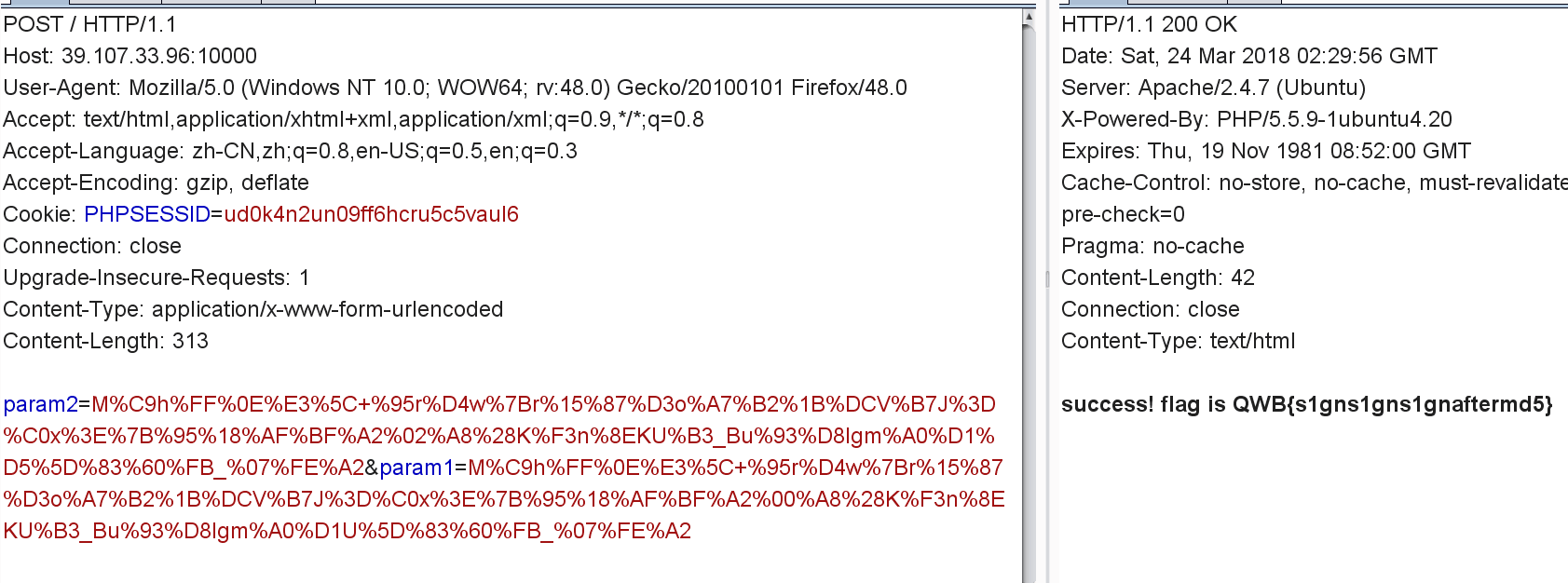# 题目备份#

https://github.com/jas502n/2018-QWB-CTF

stream

# 奇怪的心路~#

streamgame3.py

 `````` 1 2 3 4 5 6 7 8 9 10 11 12 13 14 15 16 17 18 19 20 21 22 23 24 25 26 27 28 29 30 31 32 33 34 35 36 37 38 39 40 41 42 43 44 45 46 47 48 49 50 51 52 53 54 55 56 57 58 59 60 61 62 63 64 65 66 67 68 69 70 71 72 73 74 75 76 77 78 79 `````` ``````from flag import flag assert flag.startswith("flag{") assert flag.endswith("}") assert len(flag)==24 # flag共24个字符，形如 /flag{.{18}}/ # 中间18个字符就是我们想寻找的lfsr初始状态 def lfsr(R,mask): """定义了一个lfsr的状态变化规则 输入是寄存器当前存储的状态信息和一个掩码， 两者经过一定运算得到寄存器下一个状态并输出 """ output = (R << 1) & 0xffffff #将当前值左移一位，并舍弃超出 24bit 的部分 #这就是线性反馈移位寄存器的 `移位` 部分 #可以理解为 output = (R * 2) % 0xffffff i=(R&mask)&0xffffff #将当前值和掩码按位与，只使用某些固定位的信息 #如对于 R3_mask=0x100002 ，只使用当前值左起第四位和右起第二位 #对比线性函数的定义，发现0x100002的每一位对应的就是f中的a1,a2,...,a24， #其中a4=a23=1,a1=a2=a3=a5=...=a22=a24=0 #而R的每一位对应的是b1,b2,...b24 #那么 `R&mask` 就将f中用于异或的每一项都求出来并保存在i的每一位中 #（我认为 `&0xffffff` 是多余的，R和mask都总在24位以内） lastbit=0 # lastbit实际上是函数中的a0 while i!=0: lastbit^=(i&1) i=i>>1 # 这个循环用于取出i中的每一位并完成异或操作，i中有奇数个1则lastbit为1，反之为0 # 得到的lastbit就是【寄存器当前状态的线性函数】f的函数值 # lastbit就是所谓的 `线性反馈` ，它与寄存器当前状态线性相关， # 并作为输入位，用以产生寄存器的下一个状态 output^=lastbit # 产生寄存器的下一个状态 return (output,lastbit) def single_round(R1,R1_mask,R2,R2_mask,R3,R3_mask): """完成三个线性反馈移位寄存器的一次状态变换 并将三个反馈进行简单运算（x1x2 ^ x2'x3）后返回 --【我现在明白过来了，我在 http://findneo.github.io/180325QWBWP/#streamgame3 中提到的一个失败的尝试为什么会失败，：） 就是因为最后文件中保存的每个bit都只是这个简单运算的结果 而这个简单的运算导致了每个single_round中反馈的信息 从3个bit降到了1个bit】-- """ (R1_NEW,x1)=lfsr(R1,R1_mask) (R2_NEW,x2)=lfsr(R2,R2_mask) (R3_NEW,x3)=lfsr(R3,R3_mask) return (R1_NEW,R2_NEW,R3_NEW,(x1*x2)^((x2^1)*x3)) R1=int(flag[5:11],16) R2=int(flag[11:17],16) R3=int(flag[17:23],16) assert len(bin(R1)[2:])==17 assert len(bin(R2)[2:])==19 assert len(bin(R3)[2:])==21 R1_mask=0x10020 R2_mask=0x4100c R3_mask=0x100002 for fi in range(1024): print fi tmp1mb="" for i in range(1024): tmp1kb="" for j in range(1024): tmp=0 for k in range(8): (R1,R2,R3,out)=single_round(R1,R1_mask,R2,R2_mask,R3,R3_mask) tmp = (tmp << 1) ^ out tmp1kb+=chr(tmp) tmp1mb+=tmp1kb f = open("./output/" + str(fi), "ab") f.write(tmp1mb) f.close() ``````

solution

 `````` 1 2 3 4 5 6 7 8 9 10 11 12 13 14 15 16 17 18 19 20 21 22 23 24 25 26 27 28 29 30 31 32 33 34 35 36 37 38 39 40 41 42 43 44 45 46 47 48 49 50 51 52 53 54 55 56 57 58 `````` ``````#coding:utf8 import itertools # 因为掩码的原因，大大降低了计算的复杂度。我们可以在每次规约运算时只遍历影响结果的位，而忽略其他将被掩码忽略的位。 # 这样实际上复杂度从每一次规约都有2**（17+19+21）=2**57约10**18种可能降低到了每一位约大概2**8种可能！ # 对我来说，编程实现的难点在于如何控制只遍历影响结果的位，一种是在原来的基础上加一个判断，判断是否该情况已被考虑，这应该不合适。 # 另一种就是用一个list装R的每一位 # 再有就是手动组合三个部分的每次反馈 def lfsr(R,mask): output = (R << 1) & 0xffffff i=(R&mask)&0xffffff lastbit=0 while i!=0: lastbit^=(i&1) i=i>>1 output^=lastbit return (output,lastbit) def single_round(R1,R1_mask,R2,R2_mask,R3,R3_mask): (R1_NEW,x1)=lfsr(R1,R1_mask) (R2_NEW,x2)=lfsr(R2,R2_mask) (R3_NEW,x3)=lfsr(R3,R3_mask) return (R1_NEW,R2_NEW,R3_NEW,(x1*x2)^((x2^1)*x3)) # lastbit=(R.count(1)%2?1:0) R*右边加一位lastbit # R1sm means R1_sub_mask R1_mask=0x10020;R1sm=[];R1sm.extend([0x10000,0x20]) R2_mask=0x4100c;R2sm=[];R2sm.extend([0x40000,0x1000,0x8,0x4]) R3_mask=0x100002;R3sm=[];R3sm.extend([0x100000,0x2]) def genAll(Rsm): # 全组合 all_iter=[itertools.combinations(Rsm,num) for num in xrange(len(Rsm)+1)] return itertools.chain.from_iterable(all_iter) def genBit(sub_mask,seq,mask): ret=0 for i in sub_mask: ret|=i done=mask if seq==0:done=0 else: for i in range(seq): done|=(mask>>i) ret&=(done^0xffffff)&(0xffffff<>(7-(level%8)))&0x1): digui(level+1,R1,R2,R3) f=open("output/0").read() digui(0,0,0,0) ``````

• In electronics, a flip-flop or latch is a circuit that has two stable states and can be used to store state information. flip-flop（触发器）或latch（锁存器）都是某种电路，都根据输入改变存储的状态信息，区别是前者当时钟有效时改变才发生，也就是同步的，后者是时钟无关的，也就是异步的。
• a shift register is a cascade of flip flops 。 移位寄存器是触发器的级联。
• In computing, a linear-feedback shift register (LFSR) is a shift register whose input bit is a linear function of its previous state.
线性反馈移位寄存器是一个移位寄存器，它的输入位是它先前状态的线性函数。
• 线性指的是齐次性（`f(αx) = αf(x) for all α`）和可加性（` f(x + y) = f(x) + f(y)`），两者在有理数域是等价的。上述定义中的线性函数实际上指的是布尔代数中的线性函数，形式上这样表述： 对于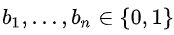，存在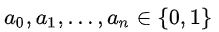，使得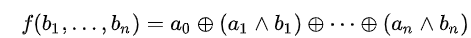，那么`f` 是一个线性函数。（其中的符号分别表示`逻辑异或``逻辑与` ，详情如下图）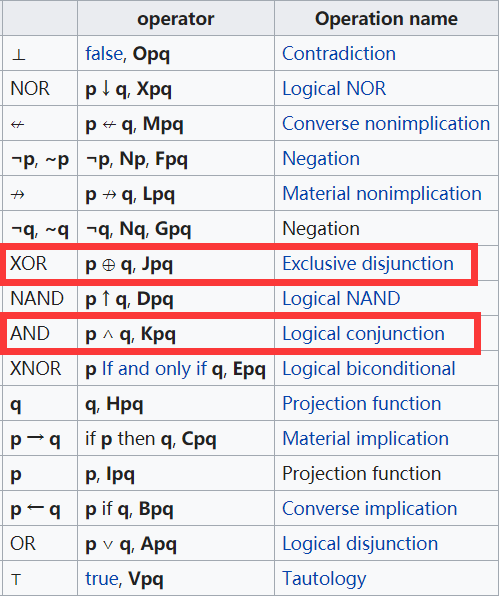`````` 1 2 3 4 5 6 7 8 9 10 11 12 13 14 15 16 17 18 19 20 21 22 23 24 25 26 27 28 29 30 31 32 33 `````` ``````def lfsr(R,mask): output = (R << 1) & 0xffffff i=(R&mask)&0xffffff lastbit=0 while i!=0: lastbit^=(i&1) i=i>>1 output^=lastbit return (output,lastbit) R1_mask=0x10020 R2_mask=0x4100c R3_mask=0x100002 import time s=''.join([bin(ord(i)).zfill(8) for i in open("output/0","rb").read()]) def handle1(start,step): for i in xrange(start,start+step): for j in xrange(2**20,2**21): R1,R3,flag=i,j,1 for offset in xrange(len(s)): R1,out1=lfsr(R1,R1_mask) R3,out3=lfsr(R3,R3_mask) if out1==out3 and out1!=s[offset]: flag=0 break if flag: print "flag{%s******%s}"%(bin(i)[2:],bin(j)[2:]) print time.asctime() exit(0) if (i-start)%10==0: print start,time.asctime(),"#" handle1(2**16,2**16) ``````